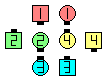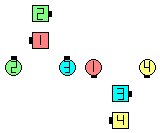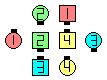Definitions of Square Dance Calls and Concepts

Index -->  Plus  |  A1  |  A2  |  C1  |  C2  |  C3A  |  C3B  |  C4  |  NOL  |
Definitions (Text Only) -->  Plus  |  A1  |  A2  |  C1  |  C2  |  C3A  |  C3B  |  C4  |  NOL  |
 Find call:

1/4 Tag \$B\$+\$i(B.

Flip Your Lid \$B\$K\$O(B 4 \$B\$D\$N0[\$J\$C\$?F0:n\$,\$"\$j\$^\$9(B:

 Center Wave \$B\$N(B Ends: \$BE,@Z\$J(B outside \$B\$N?M\$H(B Scoot Back \$B\$r\$7\$^\$9(B; Very Center: Centers Run \$B\$N<+J,\$N%Q!<%H\$r\$7(B & Circle 1/4 \$B\$r\$7\$^\$9(B (Scoot Back \$B\$r\$9\$k\$N\$G\$O\$J\$\$\$3\$H\$KCm0U(B); Outside Belle (\$B1&\$N(B 1/4 Tag \$B\$N\$H\$-(B) \$B\$^\$?\$O(B Outside Beau (\$B:8\$N(B 1/4 Tag \$B\$N\$H\$-(B): Scoot Back \$B\$N<+J,\$N%Q!<%H\$r\$7(B & Circle 1/4 \$B\$r\$7\$^\$9(B (\$BCf\$r8~\$\$\$F=*\$o\$j\$^\$9(B). \$BB>\$N(B Outside \$B\$N?M(B: Press Ahead, Trade (opposite \$B\$N?M\$H(B), & Spread \$B\$r\$7\$^\$9(B.

1/4 Tag \$B\$G=*\$o\$j\$^\$9(B.Flip Your Lid\$B\$NA0(B part-way \$B8e(B

\$B%@%s%9\$N%R%s%H(B:

• Center Wave \$B\$N(B Ends \$B\$ONY\$N(B center \$B\$N?M\$r(B \$B>/\$7\$@\$1(B\$B!!0z\$/(B (Recycle \$B\$d(B Flip Back \$B\$N\$H\$-\$N\$h\$&\$K(B) \$B\$H(B, \$B@5\$7\$\$J}\$XF0\$-;O\$a\$k\$3\$H\$N

anything & Circle (fraction) [C2]: \$BE,@Z\$J(B formation \$B\$+\$i(B. \$BA40w\$^\$?\$O(B Center 4 \$B\$,(B anything \$B\$N%3!<%k\$r9T\$\$\$^\$9(B. \$B30B&\$N(B 6 \$B?M\$,(B Swing & Circle 1/4 \$B\$N\$H\$-\$N\$h\$&\$K(B 1 position move up \$B\$7\$^\$9(B. \$B30B&\$N(B 6 \$B?M\$,(B move up \$B\$9\$kJ}8~(B (\$B;~7W2s\$j\$^\$?\$OH?;~7W2s\$j(B) \$B\$O(B anything \$B\$N%3!<%k\$r9T\$C\$?8e\$K(B Center 4 \$B\$N(B end \$B\$H\$J\$C\$??M\$N8~\$\$\$F\$\$\$kJ}8~\$K\$h\$j\$^\$9(B. & Circle 1/4 \$B\$,=*\$o\$C\$?8e(B, \$B30B&\$N(B 4 \$B?M\$OCf\$r8~\$\$\$?%+%C%W%k\$H\$J\$k\$h\$&\$K8~\$-\$rJQ\$(\$^\$9(B. \$BA4BN\$NF0\$-(B (anything \$B\$N%3!<%k\$r4^\$a\$F(B) \$B\$r3F!9(B 1/4 \$B\$4\$H\$K7+\$jJV\$7\$^\$9(B (\$BNc\$(\$P(B, fraction \$B\$,(B 3/4 \$B\$N\$H\$-\$O(B, \$BA4BN\$r(B 3 \$B2s(B). \$B\$b\$7(B fraction \$B\$,\$J\$+\$C\$?\$H\$-\$O(B, \$BA4BN\$r(B 4 \$B2s9T\$\$\$^\$9(B.Choreography for Flip Your LidComments? Questions? Suggestions?

https://www.ceder.net/def/flipyourlid.php?level=master&language=japan
04-June-2023 20:59:32